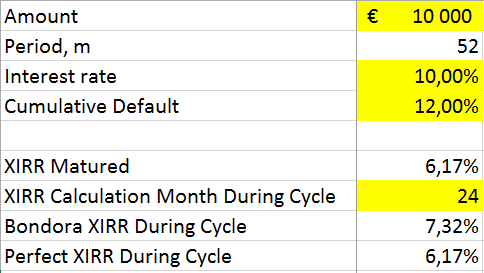# Can the net return calculation be improved?

Theoretically, it would be possible to substitute the current present value sum of scheduled future principal repayments with the sum of actual discounted future cash-flows.

However, in order to accomplish this you would need to make many assumptions about the timing and amount of future cash flows from your outstanding portfolio. You would need to estimate when and how much would current loans repay and you would have to make the same assumption also for delinquent loans. You would also need to discount these cash flows with a rate equal to actual XIRR of the fully matured investment or use future dates and payment amounts granularly in the model. In the end, one would simply arrive at a number based on a lot of assumptions that still needs to be tested in reality.

We have seen some investors build their own risk-adjusted models that simply take the outstanding principal balance and reduce this by a fraction of the overdue loans. Although this might at face-value seem like a reasonable step, it ignores the fact that outstanding loans will make both interest and principal repayments, as will recovered loans. This logic could be applied to investments with very low rates of return (where future interest payments are discounted to zero or to negative numbers) however, not to consumer loans carrying reasonable risk-adjusted interest rates.

In other words, in case one would discount overdue loans at the same time performing loans would be taken into the present value with a premium.

If you are interested in trying to establish a better model for calculating the present value, we recommend using the Historic payments and Future cashflows data exports under public or your personal data. These data sets are available for you to download from the Public Reports page. Simply using outstanding amounts is not sufficient for building a model.

The enclosed file below gives you a template example of discounting future cash flows and calculating XIRR at different points in time over the cycle of a portfolio. It also includes all the data used in the actual return calculations presented in the analyses above. Sheet ‘XIRR Calculator Example’ displays a basic model that includes some elements to include in your own analyses. Cells in yellow can be edited and white cells are fixed (please note that this example is for a 52 month term portfolio). Calculations related to ‘Bondora XIRR’ are based on the logic currently used on Bondora whilst ‘Perfect XIRR’ calculations are based on a situation where one would have perfect information available at any time during the cycle.Bondora XIRR calculation model